Graph550 0.5 Graph550 is a program to graph mathematical equations. FreewareEqPlot 1.3.22 EqPlot plots 2D graphs from complex equations. Shareware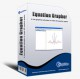Equation Grapher 2.2 Equation grapher is a free graphing calculator to graph equations. Freeware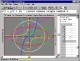Graphmatica 2.4 powerful, easy-to-use, equation plotter with numerical and calculus features Shareware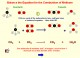Atoms, Symbols and Equations 4.0 Unique interactive multimedia Chemistry teaching software. Shareware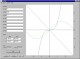MadCalc 1.1 MadCalc is a full featured graphing calculator application for Windows. SharewareGraph Paper 1.0 With Graph Paper you can create graph paper which with you can print out plain graph pages. Shareware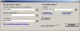PEEI 4.0.0.1 Solver of systems of partial differential equations. Freeware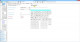ProsimGraphsPro 10.4 ProsimGraphsPro is represents a Process simulation, graphing package. SharewarePhysics Cheat Sheet 1.2 Physics Cheat Sheet is an interactive physics package that helps students solve and visualize numerous physics equations. Demo